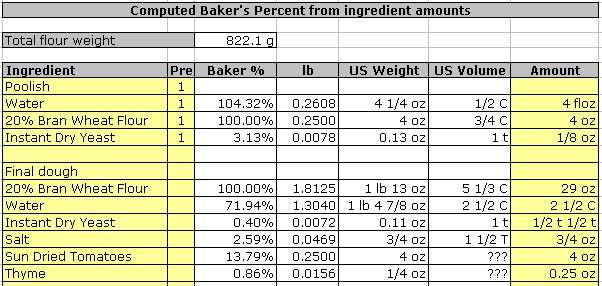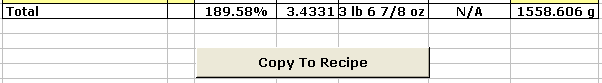• Home
• Calculator Sheets
• Dough Topics

# Introduction

You will find that there are many sources of recipes available, but many fewer for formulas (i.e. listing baker's percentages). So you will find yourself with the task of converting a recipe to a formula.

Generally speaking this task is straightforward and starts by determining the weight of the flour in the recipe, and equating that to 100%. Then express each ingredient's weight relative to that. Many US recipes (much less so in Europe, if at all) do not provide weights, but rather supply volumes (cups, tablespoons etc.).

## How to use

You start with a recipe that you find somewhere and for each ingredient you enter the description, preferment grouping code (Pre column) and the known measurement in the "Amount" column. See below for an example.This example is for Dan Leader's "Country Style Hearth Loaf with sun-dried tomatoes and thyme." I have, in most cases, entered the amount as it was specified in the original recipe (page 79). Note the use of various units, whether weights or volumes. The worksheet will accept both volume as well as weight measurements, but I suggest that if both are given, you use weights, as this will be most precise. Mixed use of weights and volumes in one entry is not supported!

Note that the water in the poolish is specified in floz. That's how it was in the recipe. The water for the final dough was also specified in floz, but to illustrate the worksheet's capabilities, I actually entered it in cups, which requires the use of a fraction. Fractions are accepted but there must not be any blanks on either side of the slash. You may also enter decimal fractions, as illustrated for the thyme ingredient. Finally, I am showing (for the instant dry yeast in the final dough) that you may enter several measurements in a row, separated by blanks. Each will be converted and the results are added together. This is very useful for recipes listing an ingredient as "1 cup plus 1 tablespoon." You would enter "1 C 1 T" for this.

Also, you may use most common abbreviations. For example "lb", "lbs", "pound", and "pounds" are all accepted and equivalent.

Once all amounts are entered you will find the computed total flour weight, in grams, on the top. At the bottom you will see something like this:Here you will find the formula total percentage, total weight in decimal pounds, total weight in pounds and ounces, and total weight in grams. Below all this is a button that, when pushed, will transfer all relevant information from this worksheet to the "Recipe" worksheet.

### Unknown ingredients

If you enter an ingredient for which there is no entry in the "Lookup" worksheet and you enter a weight, all will be fine. If, however, all you have is a volume measurement, the weight cannot be calculated and you will have to take additional action. You have several options:

1. Fill a cup measure with the ingredient an weigh it. Then, add an entry in the "Lookup" worksheet and use "CupGramsToSpecificGravity(x)" in the specific gravity column, where "x" is the weight you found.
2. Determine the weight of a cup of the ingredient by looking it up on the Internet somewhere. Again use the formula mentioned above.

Once the entry has been created, the volume amount will be correctly converted to a weight (subject to the accuracy and correctness of the value you entered). You could skip entering your findings in the "Lookup" worksheet, but it is not recommended, as each time you use this ingredient again, you will have to measure again.